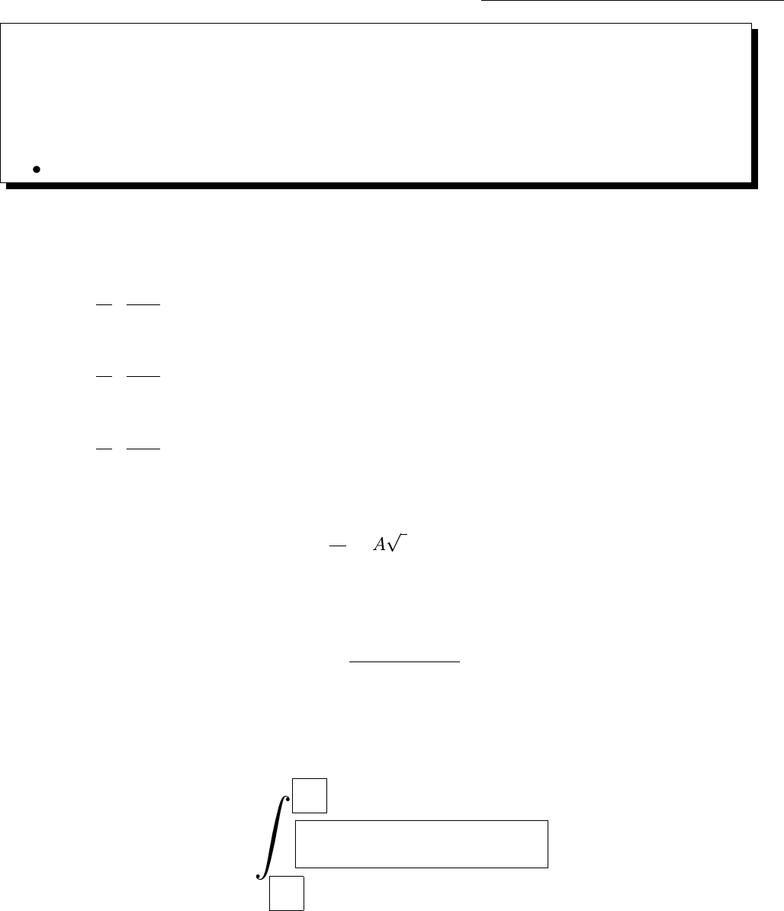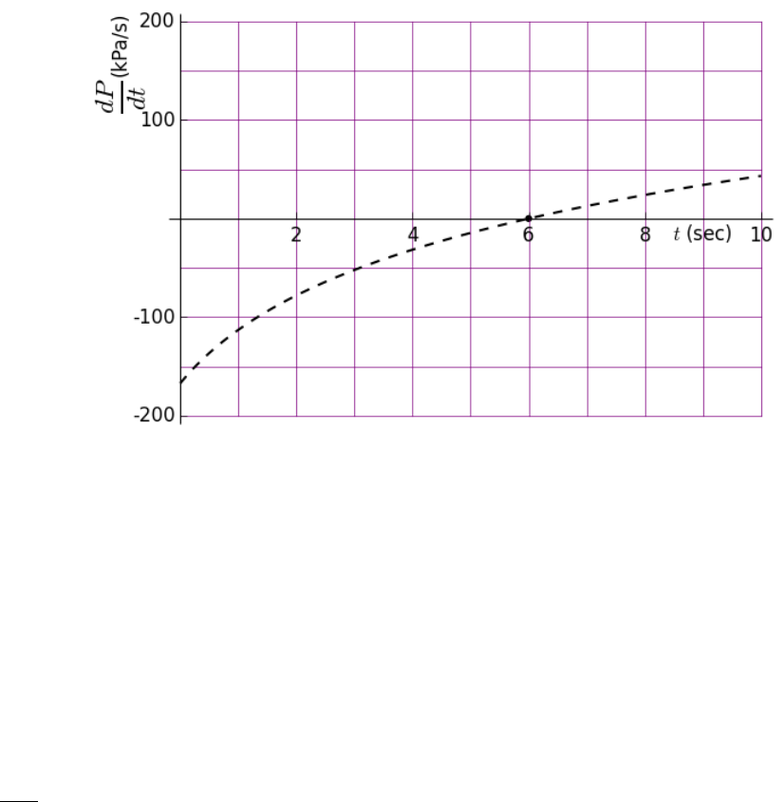Study Guides (380,000)
US (220,000)
MATH (20)
All (8)
Midterm

# MATH 165 Ball State Exam1 Review ansExam

Department
Mathematical Sciences
Course Code
MATH 165
Professor
All
Study Guide
Midterm

This preview shows pages 1-2. to view the full 6 pages of the document.Math 170 - Spring 2016 - Common Final Name:
Part 1: Short Answer
The ﬁrst six (6) pages are short answer.
You don’t need to show work.
Partial credit will be rare.
When appropriate answers must include correct units.
1. (12 points) Compute each derivative. Assume that tis the input variable and:
(a) a,fand gare constants.
d
dttfg2
a=
(b) aand gare constant and fis an unknown function of t.
d
dttfg2
a=
(c) aand fare constant and gis an unknown function of t.
d
dttfg2
a=
2. (12 points) Suppose the derivative of a function is
df
dt =AtBekt
where A > 0, B > 0 and k > 0 are unknown constants. Find a possible function f.
3. (12 points) Consider the integral
Z1
0
x2+ 1
(x3+ 3x+ 1)3dx
Use the substitution u=x3+ 3x+ 1 to transform this integral into a new integral using the variable
u. Fill in the boxes below. Do not evaluate the integral.
Z
1

Only pages 1-2 are available for preview. Some parts have been intentionally blurred.4. (16 points) The rate of change of pressure in a cylinder is given by the graph of P(t) as shown.
Which of the following are true statements? Circle all that apply.
(a) The lowest pressure in the cylinder occurs at 6 seconds.
(b) The highest pressure in the cylinder occurs at 6 seconds.
(c) ∆P > 0 on [0,1]
(d) Z10
0
P(t)dt < 0
(e) Z1
0
P(t)dt < 0
(f) d2P
dt2<0 on [0,6]
(g) The pressure is decreasing between 4 and 5 seconds.
(h) P(6) > P (3).
2
###### You're Reading a Preview

Unlock to view full version